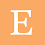Maths Class 9 Chapter 2 Polynomials

# Maths Class 9 Chapter 2 Polynomials

Important Concepts and Formulas

1.      A polynomial p(x) in one variable x is an algebraic expression in x of the form p(x) = anxn + an – 1xn – 1 + . . . + a2x2 + a1x + a0.
where a0, a1, a2, . . ., an are constants and an 0.
a0, a1, a2, . . ., an are respectively the coefficient of x0, x, x2, . . ., xn, and n is called the degree of the polynomial. Each of anxn + an – 1xn – 1 + . . . + a2x2 + a1x + a0 , with an 0, is called a term of the polynomial p(x).

2.      A polynomial with one, two and three terms are called monomial, binomial and trinomial, respectively.

3.      A polynomial of degree one is called a linear polynomial, a polynomial of degree two is called a quadratic polynomial and polynomial of degree three is called a cubic polynomial.

4.      A real number ‘a’ is a zero of a polynomial p(x) if p(a) = 0. In this case, a is also called a root of the equation p(x) = 0.

5.      Every linear polynomial in one variable has unique zero, a non-zero constant polynomial has no zero, and every real number is a zero of the zero polynomial.

6.      Remainder Theorem: If p(x) is any polynomial of degree greater than or equal to 1 and p(x) is divided by the linear polynomial (x – a), then the remainder is p(a).

7.      Factor Theorem: (x – a) is a factor of the polynomial p(x), if p(a) = 0. Also, if (x – a) is a factor of p(x), then p(a) = 0.

8.      (x + y + z)2 = x2 + y2 + z2 + 2xy + 2yz + 2zx

9.      (x + y)3 = x3 + y3 + 3xy(x + y)

10. (x – y)3 = x3 – y3 – 3xy(x – y)

11. x3 + y3 + z3 – 3xyz = (x + y + z) (x2 + y2 + z2 – xy – yz – zx)

1.2.